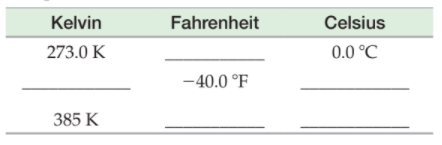×
Get Full Access to Introductory Chemistry - 5 Edition - Chapter 3 - Problem 74p
Get Full Access to Introductory Chemistry - 5 Edition - Chapter 3 - Problem 74p

×

# Complete the table.KelvinFahrenheitCelsius273.0 °C___________0.0 K_____?40.0 °F_____385ISBN: 9780321910295 34

## Solution for problem 74P Chapter 3

Introductory Chemistry | 5th Edition

• Textbook Solutions
• 2901 Step-by-step solutions solved by professors and subject experts
• Get 24/7 help from StudySoup virtual teaching assistantsIntroductory Chemistry | 5th Edition

4 5 1 247 Reviews
24
5
Problem 74P

Complete the table.Step-by-Step Solution:

Solution 74P

Step 1:

Fahrenheit(oF), Kelvin(K) and degree Celsius(oC) are the three common units of temperature.

The relationship between the three scales of temperature is given below:

C/5  =  (F-32)/9 = (K-273)/5                ------(1)

Step 2 of 2

## Discover and learn what students are asking

Unlock Textbook Solution

Enter your email below to unlock your verified solution to:

Complete the table.KelvinFahrenheitCelsius273.0 °C___________0.0 K_____?40.0 °F_____385